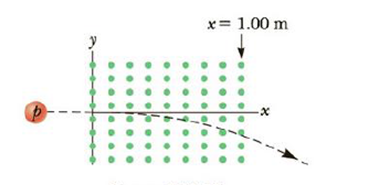Chapter 19, Problem 73AP

Chapter
Section
Textbook Problem

Protons having a kinetic energy of 5.00 MeV are moving in the positive x-direction and enter a magnetic field of 0.050 0 T in the z-direction, out of the plane of the page, and extending from x = 0 to x = 1.00 m as in Figure P19.73. (a) Calculate the y-component of the protons’ momentum as they leave the magnetic field. (b) Find the angle a between the initial velocity vector of the proton beam and the velocity vector after the beam emerges from the field. Hint: Neglect t relativistic effects and note that 1 eV = 1.60 × 10−19J.Figure P19.73

a)

To determine
The y-component of the proton’s momentum as they leave the magnetic field.

Explanation

Given info: The kinetic energy of the proton is 5.00MeV and the proton is moving in the positive x-direction. The strength of the magnetic field is 0.0500T and is along the z-direction. The magnetic field is out of the plane of the page. The magnetic field extends from x=0 to x=1.00m .

Explanation:

The arrangement of the system is shown in the figure below.

Since the magnetic field supplies the centripetal acceleration,

qvB=mv2r

• B is the magnetic field present
• v is the velocity
• q is the charge of the particle
• r is the radius of the circular path
• m is the mass of the particle

Re-arranging for the radius,

r=mvBq

The momentum of the proton will be,

p=mv

The radius will be,

r=pBq       (1)

The momentum of a particle with kinetic energy KE will be,

p=2m(KE)       (2)

Hence the radius of the proton will be,

r=2m(KE)Bq       (3)

From the figure given above the angle α will be,

α=sin1(Δxr)       (4)

• Δx is the extension of the magnetic field

Combining (3) and (4),

α=sin1(ΔxBq2m(KE)

b)

To determine
The angle between the initial velocity vector of the proton beam and the velocity vector after the beam emerges from the field.

Still sussing out bartleby?

Check out a sample textbook solution.

See a sample solution

The Solution to Your Study Problems

Bartleby provides explanations to thousands of textbook problems written by our experts, many with advanced degrees!

Get Started